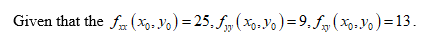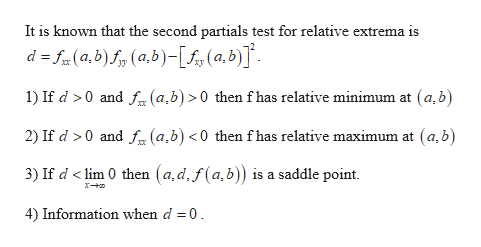# Determine whether there is a relative maximum, a relative minimum, a saddle point, or insufficient information to determine the nature of the function f(x, y) at the critical point (x0, y0). fxx(x0, y0) = 25, fyy(x0, y0) = 9, fxy(x0, y0) = 13 saddle point relative maximum insufficient information relative minimum

Question
15 views

Determine whether there is a relative maximum, a relative minimum, a saddle point, or insufficient information to determine the nature of the function f(x, y) at the critical point (x0, y0).
fxx(x0, y0) = 25, fyy(x0, y0) = 9, fxy(x0, y0) = 13
relative maximum
insufficient information
relative minimum

check_circle

Step 1help_outlineImage TranscriptioncloseIt is known that the second partials test for relative extrema is d f(a,b)f(a,b)-[£s(0,b)] 1) If d >0 and f (a,b) >0 then f has relative minimum at (a,b 2) If d 0 and f(a,b) <0 then f has relative maximum at (a,b) 3) If d < lim 0 then (a,d,f(a,b)) is a saddle point. 4) Information when d 0 fullscreen
Step 2

Obtain the value...

### Want to see the full answer?

See Solution

#### Want to see this answer and more?

Solutions are written by subject experts who are available 24/7. Questions are typically answered within 1 hour.*

See Solution
*Response times may vary by subject and question.
Tagged in

### Other